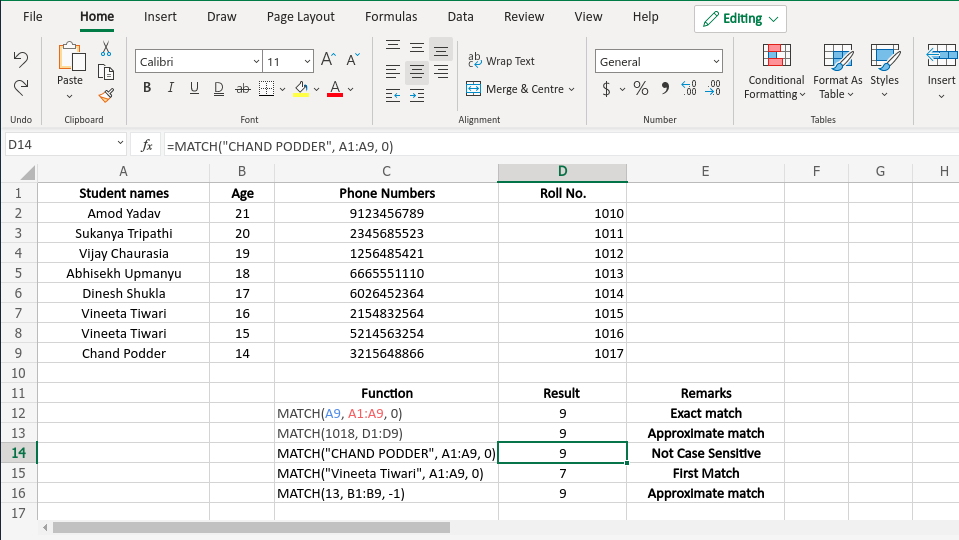Related Articles

# MATCH Function in Excel With Examples

• Last Updated : 24 May, 2021

Excel contains many useful functions and such a function is the ‘MATCH()’ function. It is basically used to get the relative position of a specific item from a range of cells(i.e. from a row or a column or from a table). This function also supports exact and approximate match like VLOOKUP() function. Generally, MATCH() function is used with the INDEX() function.

With the help of the MATCH() function, the user can get a relative position of a specific element from a table or range of cells. Relative position means the position of the element in the row or column where the MATCH() function is searching for the element. Precisely, this function helps us to get the position of an element in an array.

Syntax:

`=MATCH(lookup_value, lookup_array, [match_type])`

Here, the [match_type] value denotes if the user wants an exact match or approximate match.

Arguments:

• lookup_value (Required): It is the value(text or number or logical value or a cell reference that contains number, text or logical value) that the user wants to search. This argument must be provided by the user.
• lookup_array (Required): This argument contains the range of cells or a reference to an array where the MATCH() function will try to find out the lookup_value. Again this argument must be provided by the user.
• [match_type] (Optional): This is an optional argument. This value maybe 1, 0 or -1. If the value is 0 the user wants an exact match.  If the value is 1 MATCH() function will return the largest value that is less than or equal to lookup_value and if it is -1 the function will return the smallest value that is greater than or equal to lookup_value.

Note: If [match_type] argument is 1 or -1 then the lookup_array must be in a sorted order(ascending for 1 and descending for -1) and if the argument is not provided by the user the value becomes 1 by default.

Return Value: This function returns a value that represents the relative position of the lookup value in a range of cells.

Examples:

The example of the MATCH() function is given below. The names used in the list are only for example purposes and these are not related to any real persons.

The above list is used for example.

Below is the output screenshot of the Excel sheet.Output Screenshot

Attention reader! Don’t stop learning now. If you are an Excel beginner (or an intermediate) and want to learn Excel, Geeksforgeeks brings the perfect course for you to start, Diving Into Excel

My Personal Notes arrow_drop_up